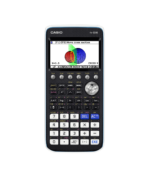Teaching Probability Distributions Archives - Casio Calculators

# Teaching Probability Distributions

Displaying Resources

FilterTeach Leaflet
fx-CG50 A-Level IB
Hypothesis Testing with the Normal Distribution

The resource consists of three learner worksheets to introduce the hypothesis te...Teach Leaflet
fx-CG50 A-Level IB
Normal Distribution Probability

The resource is an introductory pair of exercises that introduce Normal distribu...Teach Leaflet
fx-CG50 A-Level IB
Binomial Distribution Critical Regions

This teaching resource focuses on finding the boundary of a region for which the...Teach Leaflet
fx-CG50 A-Level IB
Distribution of the Sample Mean

This investigation leads learners to create samples from a Normal distribution u...Teach Leaflet
fx-CG50 A-Level IB
Binomial Distribution Probability

This activity is a pair of introductory exercises for calculating probabilities ...